## Cumulative Distribution Function From a Probability Mass Function and Vice Versa

We can construct the cumulative distribution for arandom variable where a random variable may only take certain discrete values - from the probability dens function.

Suppose the probability mass function for a random variable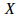is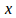0 1 2 3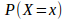0.3 0.2 0.1 0.4

For the cumulative distribution functionm we want the values of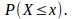We can obtain these by adding up the individual probabilities for values ofup to and including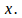We obtain0 1 2 3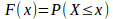0.3 0.3+0.2=0.5 0.3+0.2+0.1=0.6 0.3+0.2+0.1+0.4=1

Conversely, given a cumulative distribution function we can construct the probability mass function using the formula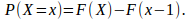From the cumulative distribution function above0 1 2 30.3 0.5-0.3=0.2 0.6-0.5=0.1 1-0.6=0.4

This returns the original probability mass function.